# MATLAB数值计算与数据分析(1)

## 基本数学函数

### 2.1.1  三角函数与双曲函数

Y = sinh(X)  %计算参量X的双曲正弦值Y

x = -pi:0.01:pi; plot(x,sin(x))

x = -5:0.01:5; plot(x,sinh(x))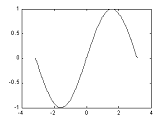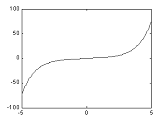Y = asinh(X)   %返回参量X中每一个元素的反双曲正弦函数值Y

x = -1:.01:1; plot(x,asin(x))

x = -5:.01:5; plot(x,asinh(x))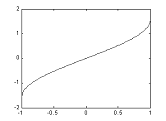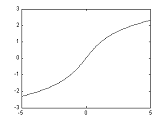Y = sinh(X)  %计算参量X的双曲余弦值Y

x = -pi:0.01:pi; plot(x,cos(x))

x = -5:0.01:5; plot(x,cosh(x))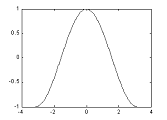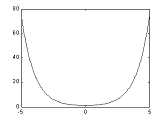Y = asinh(X)   %返回参量X中每一个元素的反双曲余弦函数Y

x = -1:.01:1; plot(x,acos(x))

x = -5:.01:5; plot(x,acosh(x))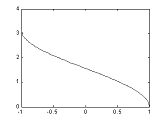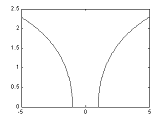Y = tanh(X)  %返回参量X中每一个元素的双曲正切函数值Y

x = (-pi/2)+0.01:0.01:(pi/2)-0.01;  % 稍微缩小定义域

plot(x,tan(x))

x = -5:0.01:5; plot(x,tanh(x))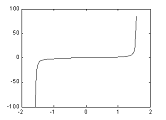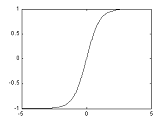Y = atanh(X)   %返回参量X中每一个元素的反双曲正切函数值Y。

x = -20:0.01:20; plot(x,atan(x))

x = -0.99:0.01:0.99; plot(x,atanh(x))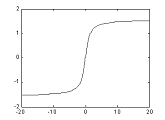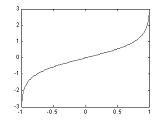Y = coth(X)    %返回参量X中每一个元素的双曲余切函数值Y

x1 = -pi+0.01:0.01:-0.01;  %  去掉奇点x = 0

x2 = 0.01:0.01:pi-0.01;  % 做法同上

plot(x1,cot(x1),x2,cot(x2))

plot(x1,coth(x1),x2,coth(x2))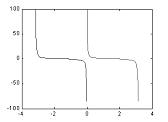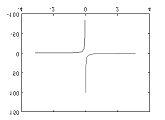Y = acoth(X)  %返回参量X中每一个元素的反双曲余切函数值Y

x1 = -2*pi:pi/30:-0.1; x2 = 0.1:pi/30:2*pi; % 去掉奇异点x = 0

plot(x1,acot(x1),x2,acot(x2))

x1 = -30:0.1:-1.1; x2 = 1.1:0.1:30;

plot(x1,acoth(x1),x2,acoth(x2))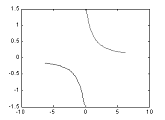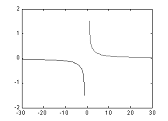Y = sech(X)  %返回参量X中每一个元素的双曲正割函数值Y

x1 = -pi/2+0.01:0.01:pi/2-0.01;  % 去掉奇异点x = pi/2

x2 = pi/2+0.01:0.01:(3*pi/2)-0.01;

plot(x1,sec(x1),x2,sec(x2))

x = -2*pi:0.01:2*pi;

plot(x,sech(x))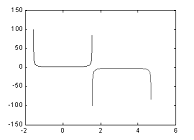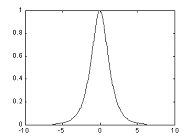Y = asech(X)   %返回参量X中每一个元素的反双曲正割函数值Y

x1 = -5:0.01:-1; x2 = 1:0.01:5;

plot(x1,asec(x1),x2,asec(x2))

x = 0.01:0.001:1; plot(x,asech(x))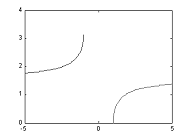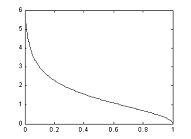Y = csch(X)    %返回参量X中每一个元素的双曲余割函数值Y

x1 = -pi+0.01:0.01:-0.01; x2 = 0.01:0.01:pi-0.01; % 去掉奇异点x=0

plot(x1,csc(x1),x2,csc(x2))

plot(x1,csch(x1),x2,csch(x2))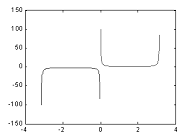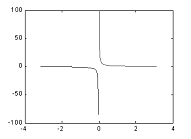Y = asech(X)   %返回参量X中每一个元素的反双曲余割函数值Y

x1 = -10:0.01:-1.01; x2 = 1.01:0.01:10; % 去掉奇异点x = 1

plot(x1,acsc(x1),x2,acsc(x2))

x1 = -20:0.01:-1; x2 = 1:0.01:20;

plot(x1,acsch(x1),x2,acsch(x2))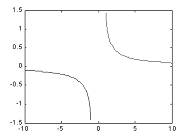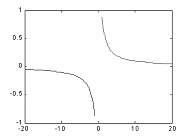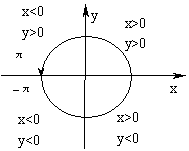z=1+2i;

r = abs(z);

theta = atan2(imag(z),real(z))

z = r *exp(i *theta)

feather(z);hold on

t=0:0.1:2*pi;

x=1+sqrt(5)*cos(t);

y=sqrt(5)*sin(t);

plot(x,y);

axis equal; hold off

theta =

1.1071

z =

1.0000 + 2.0000i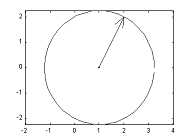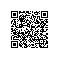使用钉钉扫一扫加入圈子
+ 订阅